# PSEB 4th Class Maths Solutions Chapter 2 Fundamental Operations on Numbers Ex 2.4

Punjab State Board PSEB 4th Class Maths Book Solutions Chapter 2 Fundamental Operations on Numbers Ex 2.4 Textbook Exercise Questions and Answers.

## PSEB Solutions for Class 4 Maths Chapter 2 Fundamental Operations on Numbers Ex 2.4

1. Find the product of the following :

Question 1.
41 × 4
Solution: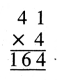Question 2.
25 × 36
Solution: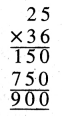Question 3.
445 × 22
Solution: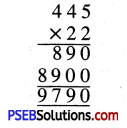Question 4.
269 × 36
Solution: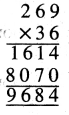Question 5.
368 × 19
Solution: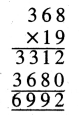Question 6.
145 × 68
Solution: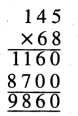Question 7.
150 × 59
Solution: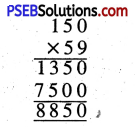Question 8.
4639 × 2
Solution: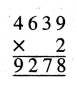Question 9.
1569 × 6
Solution: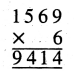Question 10.
1179 × 8
Solution: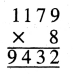Question 11.
1988 × 5
Solution: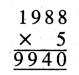Question 12.
5000 × 2
Solution: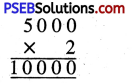Question 13.
303 × 31
Solution: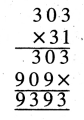Question 14.
425 × 17
Solution: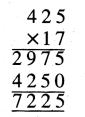Question 15.
706 × 12
Solution: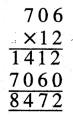Question 16.
308 × 28
Solution: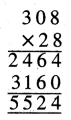2. Find product using expanded notation method:

Question 1.
52 × 7
Solution:
52 × 7 = (50 + 2) × 7
= 50 × 7 + 2 × 7
= 350 + 14
= 364.

Question 2.
63 × 4
Solution:
63 × 4 = (60 + 3) × 4
= 60 × 4 + 3 × 4
= 240 + 12
= 252Question 3.
81 × 9
Solution:
81 × 9 = (80 + 1) × 9
= 80 × 9 + 1 × 9
= 720 + 9
= 729

Question 4.
123 × 5
Solution:
123 × 5 = (100 + 20 + 3) × 5
= 100 × 5 + 20 × 5 + 3 × 5
= 500 + 100 + 15
= 615

Question 5.
205 × 6
Solution:
205 × 6 = (200 + 5) × 6
= 200 × 6 + 5 × 6
= 1200 + 30
= 12303. Find product using Lattice algorithm.

Question 1.
43 × 15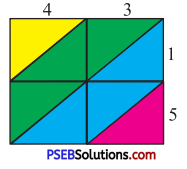Solution: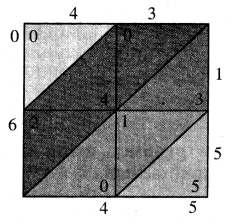Step 1: 43 × 1 (Shown in picture).
Step 2 : 43 × 5 (Shown in picture).

Question 2.
426 × 35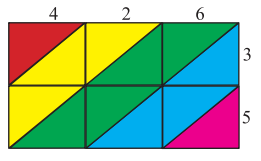Solution: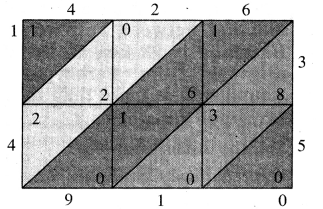Step 1: 426 × 3 (Shown in picture).
Step 2 : 426 × 5 (Shown in picture).
Step 3 : By adding diagonal elements
we got 1, 4, 9, 1, 0. which gives us no.
14910.
₹ 426 × 35 = 14910.